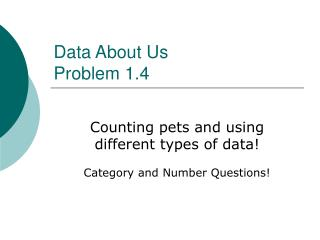Download Presentation# Data About Us Problem 1.4 - PowerPoint PPT PresentationDownload Presentation## Data About Us Problem 1.4

- - - - - - - - - - - - - - - - - - - - - - - - - - - E N D - - - - - - - - - - - - - - - - - - - - - - - - - - -
##### Presentation Transcript

1. Data About Us Problem 1.4 Counting pets and using different types of data! Category and Number Questions!

2. Categorical Data: • Values that are words that represent possible responses within a given category. • Example: What is your favorite type of soft drink? • My favorite soda is Dr. Pepper.

3. Numerical Data: • Values that are numbers such as counts, measurements and ratings. • Example: How old are you? • 11, 12, 21, 39, 67….

4. Read each of the questions below. Which questions have words or categories as answers? Which questions have numbers as answers.? • In what month were you born? • (Categorical) • What kinds of pets do you have? • (Categorical) • How many pets do you have? • (Numerical) • Who is your favorite author? • (Categorical) • How much time do you spend watching television in a day? • (Numerical) • What’s your highest score in the game Yahtzee? • (Numerical) • What color are your eyes? • (Categorical) • How many movies have you watched in the last week? • (Numerical) • How do you get to school? • (Categorical)

5. The students made tables to show the tallies or frequencies, and then made bar graphs to display the data.

6. Problem 1.4 (pg. 17) • Decide whether each questions below can be answered by using the data from the graphs the students created. If a question can be answered, give the answer and explain how you got it. If a question cannot be answered, explain why not and tell what additional information you would need to answer the question.

7. Problem 1.4 A. Which graph shows categorical data, and which graph shows numerical data? • The graph of favorite kinds of pets shows categorical data. The graph of umber of pets shows numerical data.

8. Problem 1.4 B. What is the total number of pets the students have? • 156 pets is the total number of pets the students have.

9. Problem 1.4 C. What is the greatest number of pets that any students in the class has? • 21 pets is the greatest number of pets that any student has.